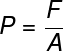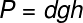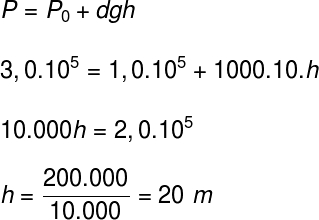# Pressure

Pressure is the physical quantity that measures the force applied perpendicularly to a surface. It is a scalar quantity , which can be calculated by the ratio of force to area. In SI units , pressure is measured in pascals , which is equivalent to newtons per square meter (1 Pa = 1 N/m²), but can also be measured in atm (1 atm = 1.1 x 10 5 Pa).

## pressure in physics

In physics, pressure is calculated by the ratio between the magnitude of a force F and an area A. Since pressure is a scalar quantity, it can be defined by its intensity and unit of measure .

The pressure is directly proportional to the intensity of the force F as well as inversely proportional to the area A. Check, below, the formula used to define it:P – pressure (Pa – pascal)

F – force (N – newton)

A – area (m²)

The formula makes sense when we relate it to sharp objects , such as needles: the area of ​​their tips is very small, so they can pierce the skin so easily, without the need to apply too great a force. This also occurs with sharp objects , such as knives, since, when sharpening them, the aim is to reduce their contact area, which increases the pressure exerted.

## hydrostatic pressure

Hydrostatic pressure is exerted by fluids in equilibrium . Fluids are substances that take the form of their containers and that can flow, such as water and air. When these fluids meet at a certain height, they produce pressures proportional to it.

Imagine a glass full of water placed on a table. The pressure this cup exerts depends on its area of ​​contact and also on the downward force it exerts. This force, in turn, depends on the weight of the cup itself and the weight of the water. The pressure from the water inside the object is hydrostatic or gauge.

The hydrostatic pressure also depends on the density of the fluid , the greater this density, the greater the pressure exerted. Note the formula used for its calculation:d – density (kg/m³)

g – gravity (m/s²)

h – height (m)

In this figure, you can see how hydrostatic pressure works Observe the distance traveled by the water that leaves each of the holes, it leaves with greater speed in the hole that is at greater depth, since the pressure exerted by it is greater at this point than at the others. If you are more curious about this topic, read our text: Hidrostatics .

## Atmospheric pressure

Earth ‘s atmospheric pressure at sea level is about 1.01325.10 5 Pa , that is, just over 100,000 newtons per square meter.

It was first measured by Italian physicist Evangelista Torricelli . To do so, Torricelli made use of a basin and a glass column, both filled with mercury. Pouring the contents of the glass column into the basin, he let the mercury flow into the container until the mercury level inside the glass tube ceased to drop.

With this he concluded that the pressure of the atmosphere corresponded to the pressure exerted by the column of mercury, which was approximately 76 cm high. It is for this reason that measurements of cmHg or mmHg have become popular and are even used to measure blood pressure .

Atmospheric pressure is particularly important when studying hydrostatics. When fluids in hydrostatic equilibrium are stored in open containers , they will be subjected to atmospheric pressure , so that their surface always remains aligned at the same height, as in communicating vessels .

Also, the pressure at the bottom of the container, called the absolute pressure , will be the sum of the hydrostatic pressure and the atmospheric pressure. The formula shown below, which is used to calculate absolute pressure, relates atmospheric pressure (P 0 ) to hydrostatic pressure and is called Stevin’s law or theorem , check:0 – atmospheric pressure (1.01.10 5 Pa or 1 atm)

As with hydrostatic pressure, atmospheric pressure varies. According to the height, as we go up, in relation to the sea level, it decreases, since the air around us becomes more and more rarefied. For comparison, the atmospheric pressure at the peak of Everest, the highest point on Earth , is about 250 mmHg , or 0.3 atm, approximately.

## Blood pressure

Blood pressure is exerted by the blood on the blood vessels. A healthy blood pressure is between 120 mmHg and 80 mmHg . Since blood pressure is greater than external atmospheric pressure, blood can travel through the circulatory system and return to the heart without the vessels collapsing.

## Exercises solved under pressure

Question 1) (Enem) One of the environmental problems experienced by agriculture today is soil compaction, due to the intense traffic of increasingly heavy machines, reducing crop productivity. One of the ways to prevent the problem of soil compaction is to replace tractor tires with tires that are more:

a) wide, reducing pressure on the ground.

b) narrow, reducing the pressure on the ground.

c) wide, increasing the pressure on the ground.

d) narrow, increasing the pressure on the ground.

e) high, reducing the pressure on the ground.

Template : letter a

Resolution:

To avoid soil compaction, it is necessary to reduce the pressure exerted on it, therefore, a possible solution is to use wider tires, which increase the contact area of ​​the machines with the soil.

Question 2) (Enem) To carry out an experiment with a PET bottle filled with water, the side of the bottle was pierced in three positions, at different heights. With the bottle capped, the water did not leak through any of the holes, and, with the bottle uncovered, the water flow was observed, as illustrated in the figure. How does atmospheric pressure interfere with the flow of water, in situations with the bottle capped and uncapped, respectively?

a) Prevents the exit of water, as it is greater than the internal pressure; does not change the flow velocity, which only depends on the pressure of the water column.

b) Prevents the exit of water, as it is greater than the internal pressure; changes the flow velocity, which is proportional to the atmospheric pressure at the height of the hole.

c) Prevents the entry of air, as it is lower than the internal pressure; changes the flow velocity, which is proportional to the atmospheric pressure at the height of the hole.

d) Prevents the exit of water, as it is greater than the internal pressure; regulates the flow rate, which only depends on atmospheric pressure.

e) Prevents the entry of air, as it is lower than the internal pressure; does not change the flow velocity, which only depends on the pressure of the water column.

Template : letter a

Resolution:

When the bottle is closed, there is no action of atmospheric pressure on the fluid. Since the hydrostatic pressure relative to the height of the fluid is less than atmospheric pressure, no water will flow from the leaky container. When the bottle is open, the atmospheric pressure adds to the hydrostatic pressure, causing the fluid to be expelled with greater speed.

Question 3) (Eear 2018 – adapted) The value of pressure recorded at the surface of a lake is 1.0 x 10 5 Pa, which corresponds to 1 atm. A diver finds himself in this lake at a depth at which he perceives a pressure of 3 atm. Knowing that the density of the water in the lake is 1000 kg/m³ and that the magnitude of the acceleration of gravity at the location is 10 m/s², at what depth, in meters, in relation to the surface, is this diver?

a) 20 m

b) 40 m

c) 30 m

d) 10 m

Template : letter a

Resolution:

To find the depth at which the diver is, just do the calculation using Stevin’s theorem, note:Based on the data informed by the statement, the diver is at a depth of 20 m in relation to the surface.

Check Also
Close